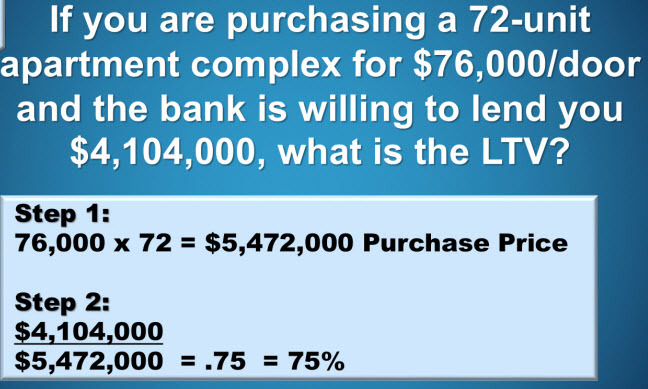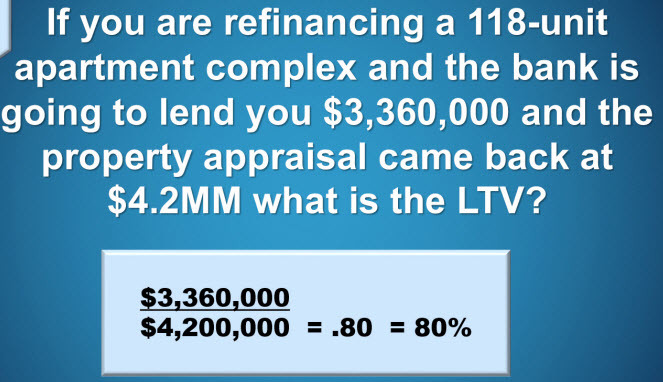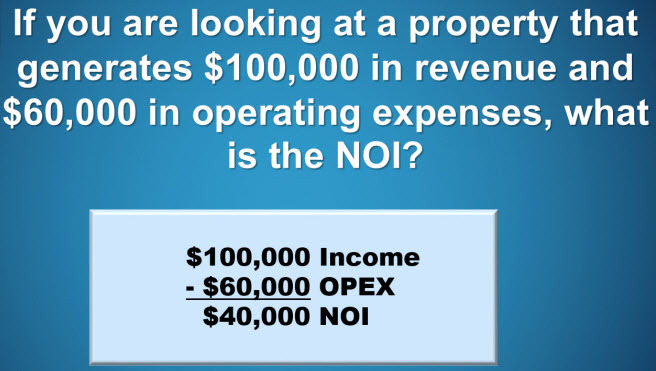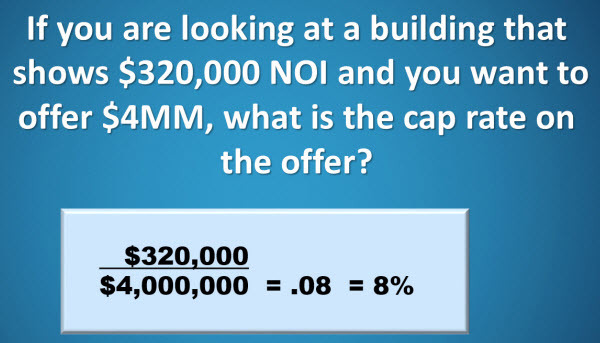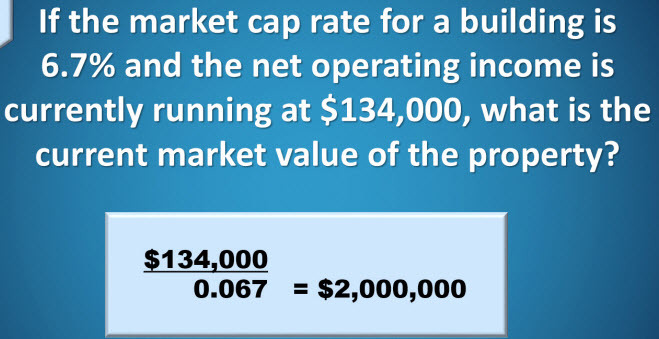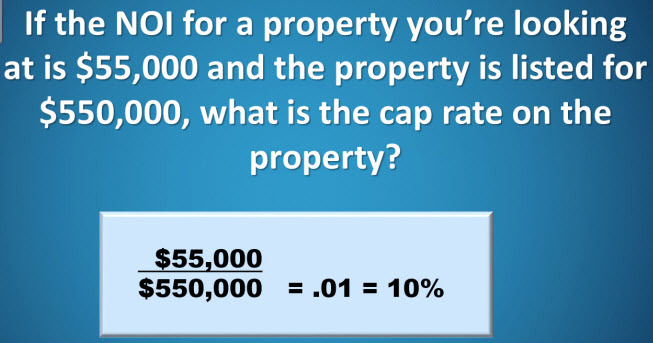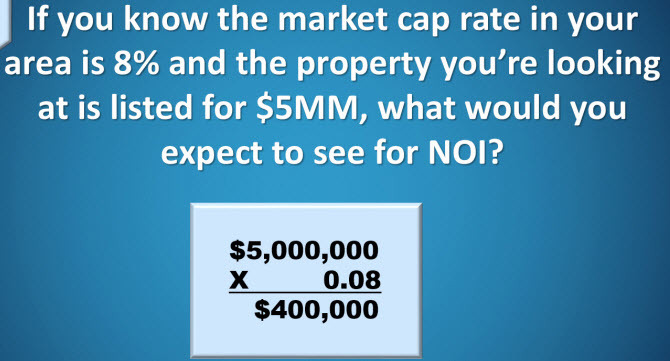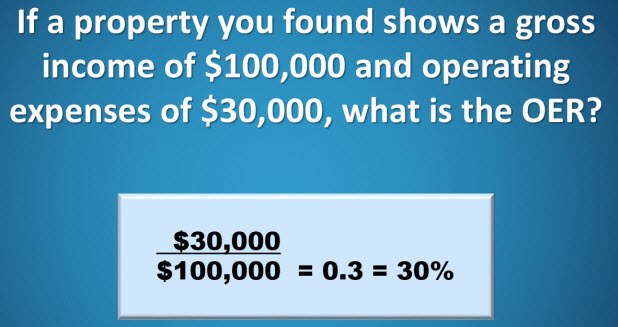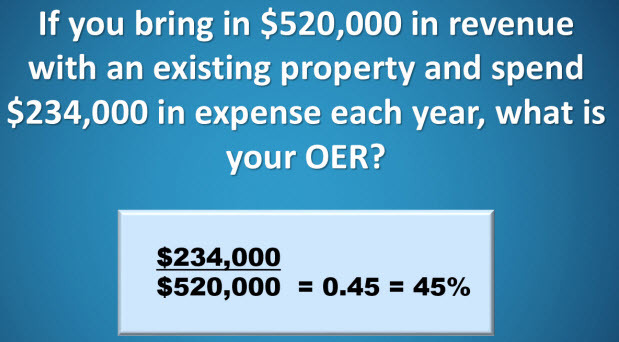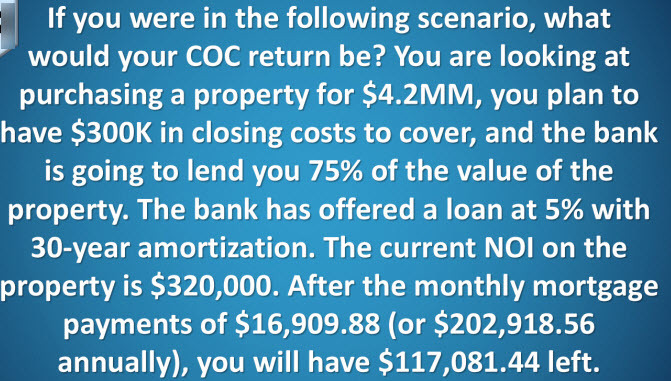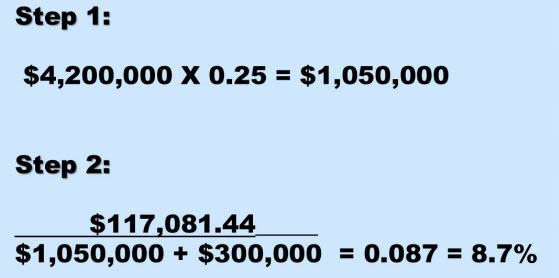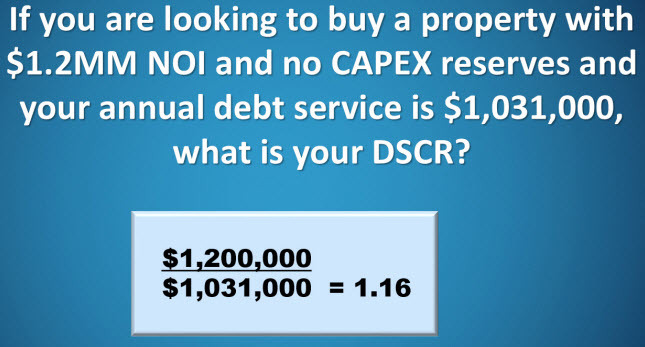# Understanding Value

Here are the main 3 metrics and how it is important to:

1. Banks want a good Cap Rate
2. Private lenders and Debt partners wants DCR
3. Investors (private equity) CoC

GPI (Gross Potential Income)

The max income the rents you take in if all of the units are being rented.  This is calculated on annual basis.

VAC (Vacancy Loss)

In perfect world all units will be rented. But that is not realistic.  That is why you have a % for vacancy.  The average is 5%.  The income lost is a collection loss.  GPI x estimated vacancy rate = VAC (vacancy loss).

EGI (Effective Gross Income)

GPI – VAC = EGI

Effective gross income is defined as your total income from possible rents minus VAC and collection loss.

OI (Other Income)

Money from sources from outside of rent. Like laundry, vending machines etc.

EGI + OI=GOI

NOI (Net Operating Income)

GPI – OE (operating expense) = NOI (net operating income)

Think of this as the difference between your before taxes income and your actual take home pay.  NOI is the incomeremaining after all expenses are paid except the mortgage.  NOI is the blood, to your apartment “body.”

NOI – RRA (Replacement Reserves Account)

RRA is intended for replacement costs that wear out, like roofing, paint, etc.

NOI – RRA – DS (Debt Service)

Deb Service is the mortgage payment.

GPI – VAC = EGI + OI = GOI –OE =NOI – RRA – DS = BTCF   BTCF = Before Tax Cash Flow

Capitalization Rate (Cap Rate) = NOI/Value of Apartment = Cap Rate

Cap rates are used to help estimate the value of income properties.  It is a measure of the return on dollars invested.  It does not consider the money borrowed.  It only considers the return on investment as if you paid all cash for the investment.  For example if the NOI is 100k, the cap rate is 10%.

Cash on Cash Return   Annual before tax cash flow = Cash on Cash Return % equity

The cash on cash return is the ratio of annual before cash flow divided by the total amount of cash invested, expressed as a percentage. It differs from cap rate, when the investor considers that the investor has used leverage, i.e. they have a mortgage on the property.  Remember cap rate is the return on investment when the investor has paid all cash for an investment, cash on cash return factors in the use of leverage (borrowed money).  The investor surely want the cash on cash on cash return to be higher than the cap rate.  If the cap rate is higher than the cash on cash return, the investor, has borrowed money with a negative repayment rate.  The cash on cash return formula is extremely important because it allows the investor to determine returns after using other people’s money (OPM) to buy the investment.

Here are the most impactful formulas you must know.  Recognize how NOI is included in each formula!

1. Cap Rate = NOI/Value
2. Cash on Cash Return = NOI – Debt Service = BTCF/Owner’s Equity
3. DCR = NOI/Annual Mortgage Payment

Here is more of a break down on understanding value.

If you raise the rent to \$10 per month in a 10 unit apartment building it raises the NOI to \$12,000 per year for the building. Do the same thing for a 50 unit apartment building and that is \$60,000 increase.

• NOI = Revenue – Expenses
• Cap Rate = NOI / Price
• Value of property = NOI / Cap Rate

Step 1:  Determine NOI.

Revenue = 10 units x \$500/mo x 12 months x 90% (the reason its 90% is because of potential vacancy loss of 10%).

Revenue = \$54,000 / year

Expenses = \$27,000/ year

NOI = \$54,000 – \$27,000 = \$27,000 /year

Step 2: Determine the cap rate at this purchase price.

Cap rate = NOI/Price

NOI = \$27,000/ yr

Price = \$270,000

Cap rate  = \$27,000 / \$270,000 = 10%

Step 3: Is this a good deal? Good deal: Purchase Cap Rate > Market Cap Rate

Purchase cap rate = 10%

Market cap rate = 8.5%

10% > 8.5% yes it is good deal.

How good is it?

NOI = \$27,000 / yr

Market Cap Rate = 8.5%

Value = \$27,000 / 8.5% = \$317,000 (rounded)

Free Equity \$317,000 – \$270,000 = \$47,000

Step 4: Determine Cash flow

Expenses do not include the mortgage (known as debt service, which is paid out of the NOI).  Cash flow is the differencebetween NOI and the debt service.  There are 3 formulas

• Cash flow (\$/yr) = NOI – Debt service payments
• Cash in the deal = down payment + closing cost + Rehab Cost
• Cash on Cash Return (%) = Cash flow / Cash in the deal

Cash in the deal includes the down payment, closing costs and rehab capital needed to address the deferred maintenance.Cash on Cash Return, is where it gets really exciting and points out the power of leverage I real estate.  When you use financing (leverage) to buy a property, the cash on cash return is always greater than the cap rate.  Example, 10 unit deal and buy it for \$270,000.  If you buy it for 25% down with a mortgage at 5% interest and 25 year amortization.

Cash Flow (\$/yr) = NOI – Debt Service Payments

NOI = \$27,000 / yr

Loan Amount = 75% x \$270,000 = \$202,500

Debt Service = \$1,184/mo x 12 = \$14,208 / yr

Cash Flow = \$27,000 – \$14,208 = \$12,792 / yr

Step 5: Determine the cash in the deal and the cash on cash return

• Cash in the deal (\$) = down payment + closing costs + rehab costs
• Cash on cash return (%) = cash flow/ cash in the deal

Down payment= 25% x \$270,000 = \$67,500

Closing costs = \$8,000

Rehab costs = 0

Cash in the deal = \$67,500 + 8,000 + 0 = \$75,500

Cash on Cash Return = \$12, 792 / \$75,500 = 16.9%

More analysis….

What is the Cap Rate of the following property?

*Purchase Price = \$10M

*Annual Income = \$1.3M

*Annual Expense = \$500,000

*Debt Service = \$480,000

NOI = \$1,3000,000 – \$500,000 = \$800,000

Cap rate @10% = 800,000 / 10,000,000 = 0.08 = 8%

How will it change if the price is reduced to \$8M?

Cap rate @8% = 800,000 / 8,000,000 = 0.10 = 10%

Higher CapRate → Cheaper Property

MAIN FACTORS AFFECTING CAP RATE

1. RISK – *Higher Risk → Higher Cap Rate
2. LENDING ENVIRONMENT – *Easier to get money → Lower Cap Rate
3. LIQUIDITY IN THE FINANCIAL MARKETS – *Rising Market → Lower Cap Rate
4. TAX CODES – *Favorable code create tax shelters → Higher Demand → Lower Cap Rate
5. APPRECIATION – *When real estate prices are expected to rise, investors are willing to pay based on a lower Cap Rate

DEBT COVERAGE RATIO (DCR)

The ratio between cash flow and mortgage payment

DCR= NOI/ Debt Service

Also known sometimes as “Debt Service Coverage Ratio” (DSCR)

What is the DCR of the following property?

*Purchase Price = \$10M

*Annual Income = \$1.3M

*Annual Expense = \$500,000

*Debt Service = \$480,000

NOI = \$1,3000,000 – \$500,000 = \$800,000

DCR = NOI / Debt Service = 800,000 / 480,000 = 1.67x

CASH-ON-CASH (COC)

All in cash = Down Payment + Closing Costs + Acquisition Fee

CoC = NOI − Debt Service / All In Cash

What is the CoC Return of the following investment?

*Purchase Price = \$10M

*Annual Income = \$1.3M

*Annual Expense = \$500,000

*Debt Service = \$480,000

*Down payment (25%) = \$2.5M

*Closing costs (1%) = \$100,000

*Acquisition Fee (5%) = \$500,000

NOI = \$1,3000,000 – \$500,000 = \$800,000

All in cash = Down Payment + Closing Costs + Acquisition Fee = \$2,500,000 + \$100,000 + \$500,000 = \$3.1M

CoC = NOI – Debt Service / All in cash

CoC = 800,000  −480,000 / 3,100,000 = 320,000 / 3,100,000 = 10.3%